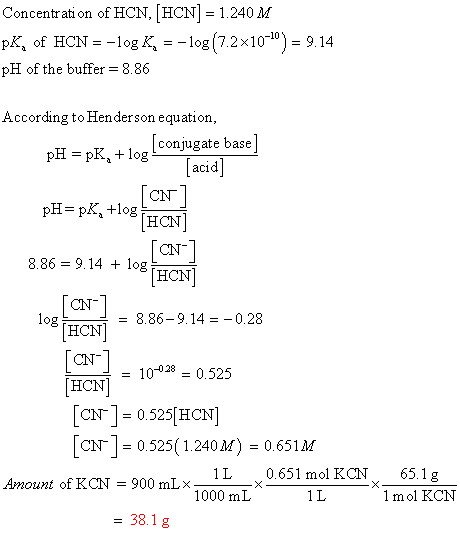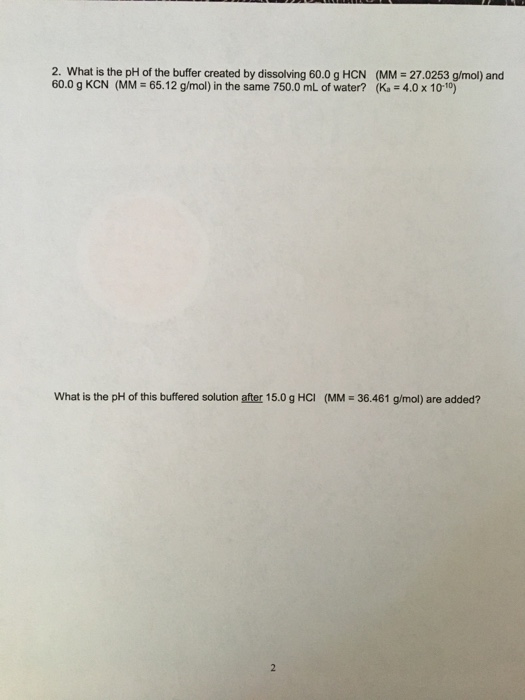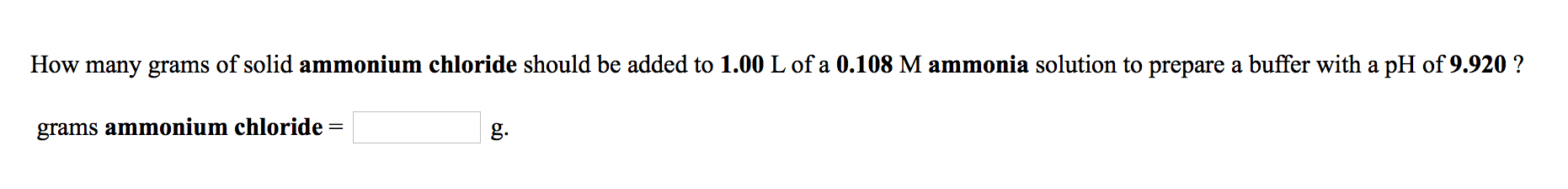Question

# How many grams of KCN (Mm = 65.1 g/mol) must be added to 900. mL of...

How many grams of KCN (Mm = 65.1 g/mol) must be added to 900. mL of 1.240-M solution of HCN in order to prepare a pH = 8.86 buffer?#### Earn Coins

Coins can be redeemed for fabulous gifts.

Similar Homework Help Questions
• ### How many grams (to the nearest 0.01 g) of NH4Cl (Mm = 53.49 g/mol) must be...

How many grams (to the nearest 0.01 g) of NH4Cl (Mm = 53.49 g/mol) must be added to 550. mL of 0.976-M solution of NH3 in order to prepare a pH = 9.15 buffer? What volume (to the nearest 0.1 mL) of 4.00-M NaOH must be added to 0.600 L of 0.300-M HNO2 to prepare a pH = 3.50 buffer?

• ### How many grams (to the nearest 0.01 g) of NH4Cl (Mm = 53.49 g/mol) must be...

How many grams (to the nearest 0.01 g) of NH4Cl (Mm = 53.49 g/mol) must be added to 750. mL of 1.188-M solution of NH3 in order to prepare a pH = 9.90 buffer?

• ### How many grams (to the nearest 0.01 g) of NH4Cl (Mm = 53.49 g/mol) must be...

How many grams (to the nearest 0.01 g) of NH4Cl (Mm = 53.49 g/mol) must be added to 750. mL of 0.928-M solution of NH3 in order to prepare a pH = 8.95 buffer?

• ### How many grams of dipotassium phthalate (242.3 g/mol) must be added to 100 mL of 0.125...

How many grams of dipotassium phthalate (242.3 g/mol) must be added to 100 mL of 0.125 M potassium hydrogen phthalate to give a buffer of pH 5.80? The Ka's for phthalic acid are 1.12 x 10-3 and 3.90 x 10-6.

• ### A. How many grams of ammonium chloride must be added to 0.80 L of 1.7 M...

A. How many grams of ammonium chloride must be added to 0.80 L of 1.7 M ammonia to prepare a pH = 10.29 buffer? B. How many milliliters of 2.9 M HCl must be added to 2.6 L of 0.17 M K2HPO4 to prepare a pH = 7.44 buffer? C. What is the pH of a solution prepared by mixing 24 mL of 0.050 M Ba(OH)2 and 39 mL of 0.44 M KOH? (This is just a mixing problem: simply...

• ### How many grams of dipotassium succinate trihydrate (K2C4H4O4·3H2O, MW = 248.32 g/mol) must be added to...

How many grams of dipotassium succinate trihydrate (K2C4H4O4·3H2O, MW = 248.32 g/mol) must be added to 650.0 mL of a 0.0420 M succinic acid solution to produce a pH of 5.867? Succinic acid has pKa values of 4.207 (pKa1) and 5.636 (pKa2).

• ### 2. What is the pH of the buffer created by dissolving 60.0 g HCN (MM =...2. What is the pH of the buffer created by dissolving 60.0 g HCN (MM = 27.0253 g/mol) and 60.0 g KCN (MM = 65.12 g/mol) in the same 750.0 mL of water? (Ks = 4.0 x 10-10) What is the pH of this buffered solution after 15.0 g HCI (MM = 36.461 g/mol) are added?

• ### How many grams of Na2SO4 (142.04 g/mol) are needed to prepare 125.0 mL of 2.50 mM...

How many grams of Na2SO4 (142.04 g/mol) are needed to prepare 125.0 mL of 2.50 mM solution of Na+? What's the concentration in ppm of Na+ in this solution?

• ### A) How many moles of sodium hydroxide would have to be added to 225 mL of...

A) How many moles of sodium hydroxide would have to be added to 225 mL of a 0.362 M hydrocyanic acid solution, in order to prepare a buffer with a pH of 9.580? B) An aqueous solution contains 0.383 M nitrous acid.   How many mL of 0.287 M sodium hydroxide would have to be added to 250 mL of this solution in order to prepare a buffer with a pH of 3.600? C) An aqueous solution contains 0.313 M ethylamine...

• ### A. B. C. How many grams of solid ammonium chloride should be added to 1.00 L...A. B. C. How many grams of solid ammonium chloride should be added to 1.00 L of a 0.108 Mammonia solution to prepare a buffer with a pH of 9.920 ? grams ammonium chloride = g. How many grams of solid sodium cyanide should be added to 1.00 L of a 0.196 M hydrocyanic acid solution to prepare a buffer with a pH of 8.528 ? grams sodium cyanide = How many grams of solid ammonium chloride should be added...